# College Physics : Electromagnetic Spectrum

## Example Questions

### Example Question #1 : Electromagnetic Spectrum

An x-ray machine emits electromagnetic photons carrying a frequency of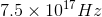. What is the approximate energy carried by each photon?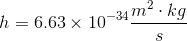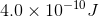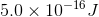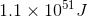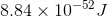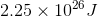Explanation:

The energy of a photon of a given frequency is determined by the equation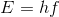, whereis Plank's constant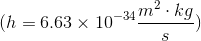So plug in Plank's constant and the frequency of the x-ray photons to get an energy of very near### All College Physics Resources